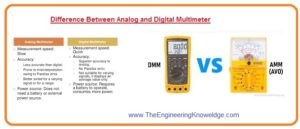Hello guys, I hope you all are doing great. In today’s tutorial, we will have a look at Difference Between Analog and Digital Multimeter. The multimeter is an electronic device that used to measure different quantities like voltage, current, resistance. There are 2 main types of multimeter first is analog and second is a digital multimeter. The main difference among them is their way by which they show output.

The analog meter shows calculated values through analog way by showing the position of a pointer on the scale. While digital meter shows the measured values in the form of numbers on the screen. In today’s post, we will have a detailed look at both meters features and compare them to find their related differences. So let’s get started with Difference Between Analog and Digital Multimeter.

#### Difference Between Analog and Digital Multimeter

Analog Multimeter

• This displays the calculated values through the deflection of the pointer.
• In this meter, an analog to digital converter does not exist.
• It used to finds the values of different electrical terms lie volts, current frequency.
• Its main component includes the function of volts, resistance, and ampere.
• It helps to solve electronic and electrical faults
• The latest meter helps to measure capacitor diodes testing
• The accuracy in output is less for this meter..
• It causes less noise.
• During operation, it gets only one input signal.
• Its price is less.
• Its physical area is larger.
• It is difficult to use.
• It calibrated through the manual way.
• It comes with the dc volts, ac volts, dc current, and different decibels calculations
• The analog meter used to measure current has a clamp for connection
• During buying the meter its measuring value must be checked
• ts main features are battery, overload protection and mirror scale
• It has overload protection with fuses.
• It has mirrored scale that used to measure temperature values.
• It comes with a bench top. That used to handle and wheels.
• Its input resistance values can be varied according to the range
• To the operation of the analog meter, there is no need of a power source. The power source is only needed when resistance is measured.
• Its circuit configuration is simple as compared to the digital meter.
• The frequency range for this meter is two kilohertz.
• Analog meters find the values of voltage, current, and resistance.

Digital Multimeter

• The output of the meter is shown in the form of digits.
• It used to measure the 2 or more than 2 electrical terms like volts resistance and current
• It mostly employed in industries
• It requires an analog to digital converter to show values.
• It has four main parts display buttons dial and input jack
• Its accuracy in the calculation as compared to the analog meter is large.
• Its size is less than the analog meter.
• Its price is high.
• It can be easily used than the analog meter.
• Its input remains constant for all ranges.
• It causes high noise.
• It needed a power source for operation.
• Its circuit is complicated than analog meter due use of ADC, LCD.
• This meter can also measure current voltage, resistance, impedance, and can test the diode and transistor.That is a detailed post about the difference between the analog multimeter and digital multimeter if you have any query ask in comments. Thanks for reading. Have a good day.